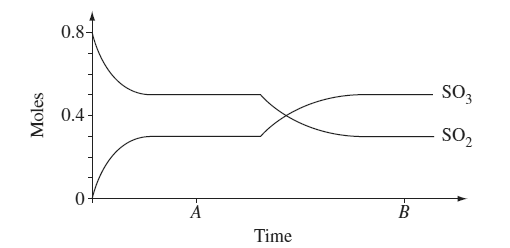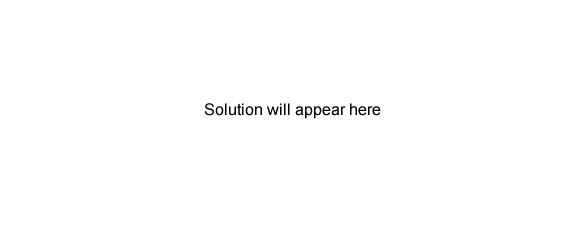Chemical equilibrium (2010 NSW HSC) 1) At room temperature 0.80 moles of SO2 and 0.40 moles of O2 were introduced into a sealed 10 L vessel and allowed to come to equilibrium.a) Write the equilibrium constant expression and calculate the value for the equilibrium constant at time A. Solution (b) Explain why a new equilibrium position was established at time B. Solution